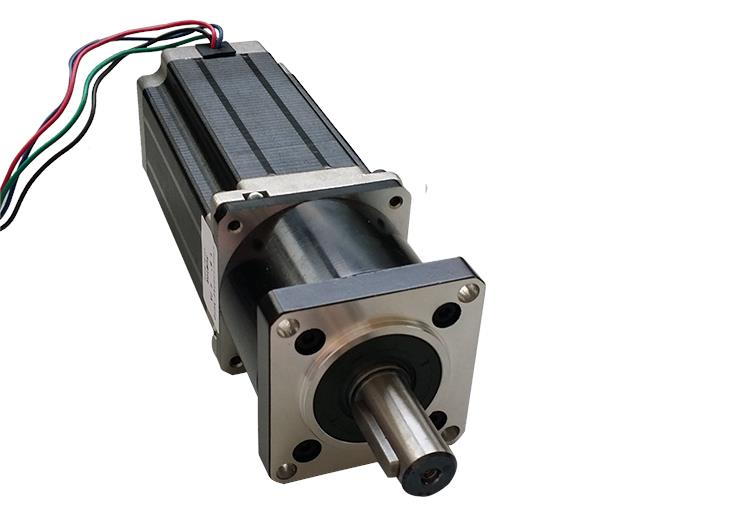# 6 Factors To Consider To Choose a DC Stepper Motor Gearbox

## 6 Factors To Consider To Choose a DC Stepper Motor Gearbox

DC stepper motor Gearbox is a very widely used reducer transmission equipment, in the selection of the following Factors need to be considered.

1. Step angle selection: the step angle of the motor depends on the requirements of the load accuracy, the minimum resolution of the load (equivalent) converted to the motor shaft, how many angles each equivalent motor should go (including deceleration). The step angle of the motor should be equal to or less than this angle. The step angle of the stepper motor on the market is generally 0.36 degrees / 0.72 degrees (five-phase motor), 0.9 degrees / 1.8 degrees (two, four-phase motor), 1.5 degrees / 3 degrees (three-phase motor), etc.

2. Static torque selection: the dynamic torque of the stepper motor is difficult to determine at once, we tend to determine the static torque of the motor first. Static torque selection is based on the motor work load, and the load can be divided into two kinds of inertial load and friction load. A single inertial load and a single frictional load do not exist.

When starting directly (generally from low speed) both loads should be considered, when accelerating starting, the inertia load is mainly considered, and when running at constant speed, as long as the friction load is considered. In general, the static torque should be 2-3 times the friction load within the good, static torque once selected, the motor seat and length will be able to determine (geometry).

3. The choice of current: static torque of the same motor, due to the different current parameters, its operating characteristics vary greatly, can be based on the moment-frequency characteristics graph, to determine the motor current.

4. Choice of type: There are two types of geared motors: cage type and wire-wound type. Cage geared motor structure is simple, easy to maintain, inexpensive, but the starting performance is poor, the general no-load or light load starting production machinery side can be selected; wire-wound geared motor starting torque, starting current is small, but the structure is complex, starting and maintenance is more trouble, only for the need for large starting torque occasions, such as lifting equipment, in addition to the need for appropriate speed control of mechanical equipment.

5. Power selection: The selection of the power of the stepper motor gearbox is determined by the production machinery, that is, by the power required by the load. The required motor power of the speed reduction motor with the following formula: motor power = torque ÷ 9550 × motor power input rpm ÷ speed ratio ÷ use factor .

6. The choice of speed: the speed of the geared motor is close to the synchronous speed, and the magnetic field speed is graded by the number of magnetic pole log P. The speed between the two grades is not available. The principle of speed selection of geared motor is to make it as close as possible to the speed of production machinery to simplify the rotating device.